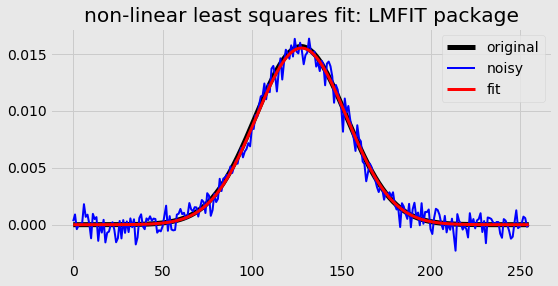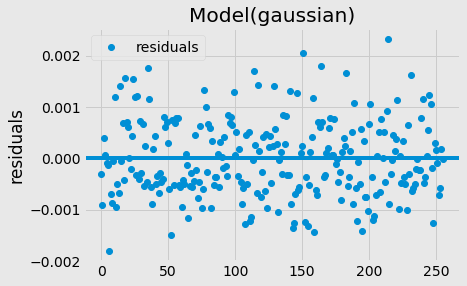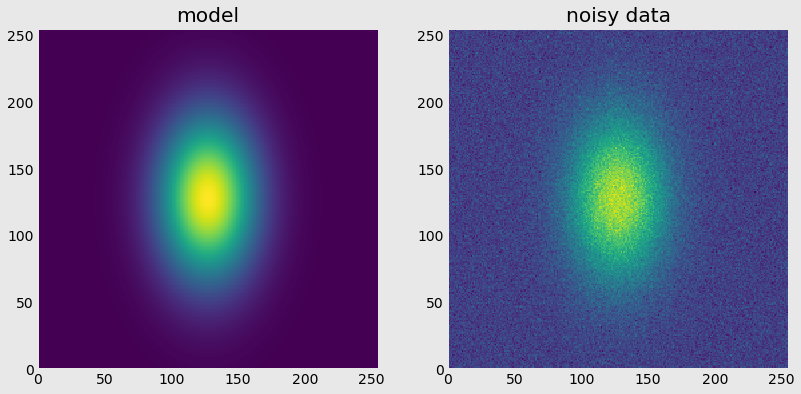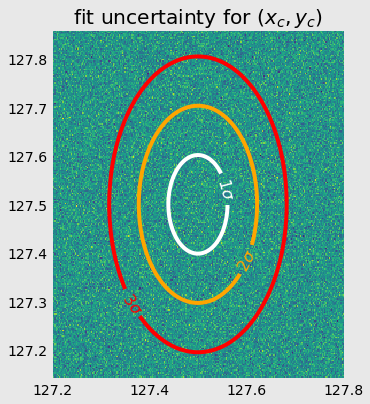# Non-linear fitting with python in 1D, 2D, and beyond…

For tasks requiring even the slightest amount of sophistication, the use of options like MS Excel becomes unrealistic. At this point, tools like MATLAB, Mathematica, or OriginLab must be employed.

An increasingly popular option within the sciences is the use of the Python programming language, particularly within the Jupyter Notebook environment, due to the very powerful methods of manipulation and inspection made available through an array of open source packages. In particular, and for our use here, the Numpy (matrix manipulation), Scipy (scientific/engineering algorithms), and Matplotlib (advanced plotting) packages offer a robust suite of analysis tools.

Non-linear least squares fitting in Python can easily be achieved with either of two options: + the curve_fit() function from scipy.optimize + the LMFIT package, which is a powerful extension of scipy.optimize

Examples using both are demonstrated below.

# Fitting in 1D

The data to be considered will be a simple Gaussian distribution of amplitude,, centered at, and standard distribution,:## Method 1: using the scipy.optimize package

The scipy package is easy to use and performs very well, but only returns limited information. Most of the time, though, it’s exactly what you want: the fit values and covariance matrix. The diagonal elements of the covariance matrix equal the variance of each fit parameter, which can be used to calculate the fit parameter uncertainties,.Unfortunately, any goodness of fit (,, etc.) is not returned, but it can easily be calculated:## Method 2: using the LMFIT package

Under the hood, the LMFIT package actually uses scipy.optimize, but adds more advanced functionality for fit options and gives immediate access to fit perfomance and data statistics (see below). Althoughand theare automatically calculated,is not. Again, it’s a trivial matter to calculate it.

You might notice here that the parameters can have several conditions placed on them in order to constrain the fit routine, such as: min and max bounds, ability to fix a value, or forcing a parameter to adhere to an analytic expression. Use of these is covered in the LMFIT documentation.# Fitting in 2D

Now, let’s look at a more challenging example – least squares fitting over multiple independent dimentions. Why is this challenging? The curve fitting algorithm we’re using here only accepts 1D arrays and expects the fitting function to only return a 1D array. But this won’t stop us.. We’ll just pass a 1D array of ND array elements (here, N = 2) and use this to build our ND fitting function, flattening the output back down to 1D for the function return.

This time, the data to be considered will be a 2D Gaussian (normal) distribution, without any assumption that variance in theanddirections are equal ():## Method 1: using the scipy.optimize package

You can already see the relavant changes in the definition of the distribution function. It takes in a 2D field ofandvalues, produces a 2D array of normally distributed points, and the the return flattens everything out using np.ravel(). Let’s first plot an ideal version of this function and then produce a slightly noisy version we can apply our fit routine towards.Let’s then take this noisy data, and apply the curve_fit() routine. This part looks a lot like the 1D case! Just notice the np.ravel() in the call to curve_fit().

If we are simply interested in showing the standard deviations from the mean, we’ll need to plot the waist contours,,,, etc. This just shows the probability coverage of our function and doesn’t take advantage of the fitting we’ve just done, but it does allow us to show how to create contours on a 2D plot, which we’ll need for the next part.Now, let’s actually display the standard deviation in the mean, as a result of our fitting procedure. The error here was quite small (~0.03%), which means less than a pixel in diameter in the image above. We’ll have to rescale this image to zoom in for a view of the fit uncertainty on the center.Wow.. we’ve narrowed the position of the distribution peak down so much that there’s hardly any variation in the data on this scale. This just goes to show: an excellent fit result starts with excellent data (and a correct choice of analytical model).

## Method 2: using the LMFIT package

Again, LMFIT is extraordinarily easy to use. All is exactly the same as for the 1D case, only with the np.ravel(). In each of these cases, the major changes we’re making is to the fit function itself so that flattened data can be compared to a flattened model.

# Fitting in ND…

As you can see, the entire trick hinges on this:

1. create your multidimensional fitting function, but flatten the output to 1D;
2. flatten your multidimensional data to 1D;
3. employ the fit routine as you would normally for the 1D case, given the package you’re working with.

With this as your algorithm, you can scale the 2D procudure outlined above in a fairly straightforward manner. It would be interesting to see the limits of this proceedure, though. I know the folks over at Big Data have some slick tricks for doing things like this. I’m just curious how much of it essentially comes down to applying these same procedures after taking an out-of-memory data set and applying principle component analysis on it as a sub-selection step.

IN any case, there it is… Not too terribly difficult after all. As long as you have correctly chosen your model and are able to faithfully code it up, you should now be able to quantify how well measured reality matches mathematical expectation.

1.Filippo

Hi. I wrote a routine to fit a 1D absorption line using the LMFIT technique you described. Actually I ‘m having some troubble with chi squared output (and with errors estimation on the fitted parameter…) I see in your example that you obtain a very low chi squared , too…Actually it’s the same situation I encountered…too low chi squared. I red on LMFIT documentation that the fit must be weighted to obtain a correct estimation of statistical parameter. Actually I was wondering if you have some ideas on how to do it (if I weight my fit on the error bar of data points, the chi squared reduces more…)

•Kipp van Schooten

Thanks for the notice on LMFIT’s use of chi squared. I’ll look into it and try to find the documented issue you’re having.

2.Dan

For fitting in 1D, if you had an unknown distribution of x values, for example one pixel through time, how would you define the guess values? In my case, some pixels will have a sharp increasing amplitude (change over time) and others remain flat (no change). My overall objective is to classify each pixel based on its curvature. Thank you.

•Kipp van Schooten

Without knowing reasonable bounds of the feature you wish to fit prior to fitting, it is really hard to define one set of guess values that are appropriate for all situations. If the feature you are fitting is fairly consistent with your model, but shows up sporadically (random times in ‘x’), you could first use a peak-finding routine to define the feature’s position along x-axis. (It sounds like your features-of-interest are peaks. If not, there are other search routines based on zero-crossings, fourier features, or even wavelet analysis.) Once the position in ‘x’ is known, then perform a local fit around the feature using a consistent set of guess values.

If the amplitude of your features/peaks is not roughly constant (varies by >100%), you could still use this peak finding method by adjusting your guess values based on the data around your newly found peak position. For example, if your model was a gaussian (as above), then the data at the x-position returned by your peak-finding routine will allow you to scale the amplitude used in your guess values.

•Dan

This makes sense. And to follow up, is there any significant benefit to using the optimize function over scipy’s UnivariateSpline in my case? It seems like the spline fit is flexible enough given the smoothing parameter and it does not require guess values. Thank you.

•Kipp van Schooten

It depends on what you’re after. Spline interpolation is a data smoothing method and not actually a fit to the data. A fitting routine compares your data to some analytical model/distribution (Ex: gaussian distribution) – as long as you can justify the use of that distribution for your data, then the fit parameters give insight to the nature of your data source or measurable. The comparison of your data to a model also allows you to determine uncertainties and apply error bars.

Using interpolation correctly can be tricky, so be careful. It makes noisy data look really nice, but it basically amounts to fabricating data since it is making up data points that you didn’t actually measure. If you are interested in smoothing noisy time-series data, then Fourier filtering is a much better option since you can rationally describe how you treat your data. If you understand the signal you are searching for, then apply a filter which attenuates any frequency components *not* contained in your signal. roadrunner66 shows a nice, concise example of this in his answer on stackoverflow. Best of luck with your data analysis!

•Dan

Thank you very much!

3.paul

Thanks for the example code.

Try this:
GRID_WIDTH = 256
GRID_HEIGHT = 255

x = np.arange(GRID_WIDTH)
y = np.arange(GRID_HEIGHT)

The image will be skewed; somewhere, you are applying the sizes of x and y incorrectly. It looks like np.outer(x, y) should be np.outer(y, x)